﻿ 成渝城市群城镇化质量时空演变

# 成渝城市群城镇化质量时空演变Temporal and Spatial Evolution of Urbanization Quality of Chengdu-Chongqing Urban Agglomeration

Abstract: By constructing the urbanization quality index system and using the entropy method to compre-hensively evaluate the urbanization quality of the Chengdu-Chongqing urban agglomeration in four periods, combined with the ArcGIS spatial autocorrelation and hotspot analysis, the paper explores the spatial pattern evolution of urbanization quality. The research shows that: the overall level of urbanization quality of Chengdu-Chongqing urban agglomeration has increased year by year, but the two-level differentiation is serious, and the quality of urbanization is still dominated by the development of low-level and medium-level. From 2004 to 2016, the average urbanization quality rose from 0.16 to 0.20. The highest urbanization quality was Chengdu (0.93) and Chongqing’s main city (0.73). The lowest was Liangping County (0.09), which was less than Chengdu's tenth. There are many cities with lower quality. 2) The spatial distribution of urbanization quality is uneven, and the development difference is obvious. The high (higher) urbanization quality cities are mainly distributed in the Chengmiande area centered on Chengdu, the central area of Chuanan centered on Leshan City and Yibin City, and the main city of Chongqing. 3) The overall autocorrelation of urbanization quality is not significant, and local autocorrelation is increasingly significant. There is no obvious accumulation of high urbanization quality cities, spatial correlation is not significant, but strong spatial autocorrelation is formed locally. The spatial variation of cold hotspots is obvious, and the number of hotspots is gradually increasing, showing a certain spatial aggregation pattern.

1. 引言

2. 方法与数据来源

2.1. 指标体系构建和数据来源Table 1. Urbanization quality index system

2.2. 方法原理

1) 数据标准化处理：在确定权重之前需要对量纲和数量级不同的各指标进行归一化处理。根据指标值大小对系统发展贡献的利弊分别进行正向处理和负向处理。

${X}_{ij}^{\text{'}}=\left({X}_{ij}-\mathrm{min}\left\{{X}_{j}\right\}\right)/\left(\mathrm{max}\left\{{X}_{j}\right\}-\mathrm{min}\left\{{X}_{j}\right\}\right)$ (1)

${X}_{ij}^{\text{'}}=\left(\mathrm{min}\left\{{X}_{j}\right\}-{X}_{ij}\right)/\left(\mathrm{max}\left\{{X}_{j}\right\}-\mathrm{min}\left\{{X}_{j}\right\}\right)$ (2)

2) 计算第 $i$ 年份第 $j$ 项指标值的比重：

${Y}_{ij}={X}_{ij}^{\text{'}}/\underset{i=1}{\overset{m}{\sum }}{X}_{ij}^{\text{'}}$ (3)

3) 计算第 $j$ 项指标的熵值：

${e}_{j}=-k\underset{i=1}{\overset{m}{\sum }}{Y}_{ij}\mathrm{ln}{Y}_{ij}$ ，其中 $k=1/\mathrm{ln}m$ (4)

4) 计算第 $j$ 项指标的差异系数：

${D}_{j}=1-{e}_{j}$ (5)

5) 计算第 $j$ 项指标的权重：

${w}_{j}={D}_{j}/\underset{j=1}{\overset{n}{\sum }}{D}_{j}$ (6)

6) 计算单项指标得分：

${S}_{ij}={w}_{j}×{X}_{ij}^{\text{'}}$ (7)

7) 第 $i$ 个年份的综合得分：

${S}_{i}=\underset{j}{\overset{n}{\sum }}{S}_{ij}$ (8)

2.3. 局自相关与热点区分析

Moran’s I： $I=\frac{n\underset{i=1}{\overset{n}{\sum }}\underset{j=1}{\overset{n}{\sum }}{W}_{ij}\left({x}_{i}-\stackrel{¯}{x}\right)\left({x}_{j}-\stackrel{¯}{x}\right)}{\underset{i=1}{\overset{n}{\sum }}\underset{j=1}{\overset{n}{\sum }}{W}_{ij}\underset{i=1}{\overset{n}{\sum }}{\left({x}_{i}-\stackrel{¯}{x}\right)}^{2}}Z=\frac{I-E\left(I\right)}{\sqrt{VAR\left(I\right)}}$ (9)

Getis-Ord G：(10)

${W}_{ij}$ 是衡量空间事物之间关系的权重矩阵，相邻为1，不相邻则为0。

$E\left(I\right)$$I$ 的理论值， $VAR\left(I\right)$$I$ 的方差，

Getis-Ord Gi*： $G\left(i\right)=\frac{\underset{i=1}{\overset{n}{\sum }}{W}_{ij}{x}_{j}}{\underset{j=1}{\overset{n}{\sum }}{x}_{j}}$ (12)

Getis-Ord Gi*所得值越高，则表示在给定距离下，该城市所产生的影响力越大，由此可以成为高城镇化质量发生的热点区域。通过Z-score得分对Getis-Ord Gi*进行检验，根据其值的正负大小判断城镇化质量是否存在高值或低值的空间聚集关系。

3. 城镇化质量综合评价与分析

3.1. 城镇化质量分析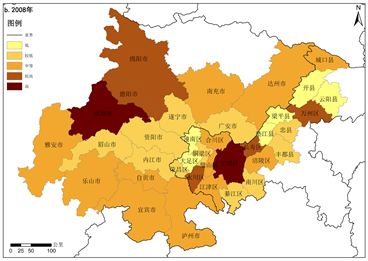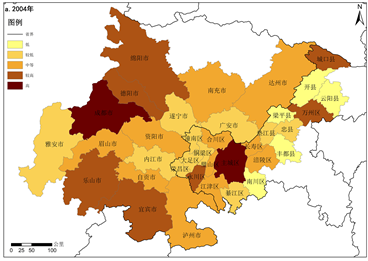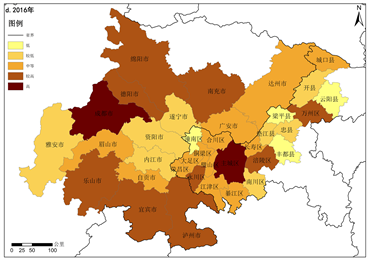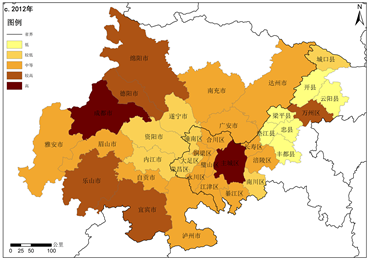Figure 1. Urbanization quality level

3.2. 空间自相关分析Table 2. Spatial autocorrelation index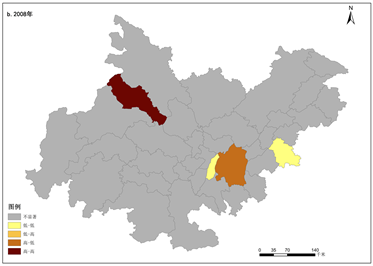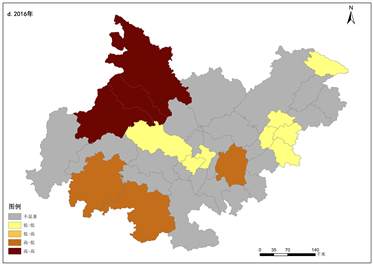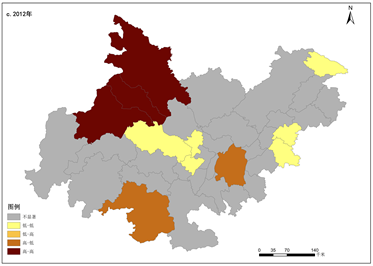Figure 2. Local spatial autocorrelation

3.3. 热点区演变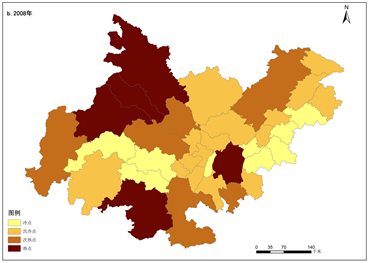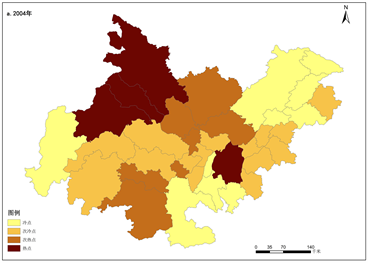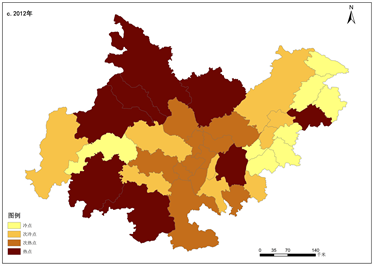Figure 3. Cold hot spot distribution

4. 结果与讨论

1) 从时间层面看，成渝城市群城镇化质量的整体水平逐年上升，但两级分化严重，城镇化质量中低水平的城市仍然占据主导地位。2004~2016年平均城镇化质量从0.16上升到0.20，绝大部分城市的城镇化质量都有不同程度的提升，但高城镇化质量指数的城市其值远大于低城镇化质量指数的城市，如2016年成都市(0.93)和重庆主城区(0.73)远大于最低的梁平县(0.09)，梁平县城镇化质量指数仅为成都市的十分之一，同时城镇化质量大于0.20的城市仅有9个，其余27个城市均低于0.20，其中梁平县和丰都县均低于0.10。

2) 从空间格局看，不同地区的城镇化质量空间不平衡，发展差异明显。高(较高)城镇化质量城市主要分布于以成都市为中心的成绵德区域、以乐山市、宜宾市为中心的川南中心区域以及重庆主城区，呈现3个区域中心并存的发展格局，同时城镇化质量提高最快的是重庆主城区，达到0.14，而最低的为城口县，仅为−0.01，差异显著。

3) 城镇化质量全局自相关不显著，局部自相关日益显著。整体来看城镇化质量高的城市没有出现明显的聚集，缺乏全局性的高值聚集中心，空间相关性小，但在局部形成较强的空间自相关，呈现空间聚集格局，较为明显的有成都市、德阳市、绵阳市形成的热点区、乐山市、宜宾市、泸州市形成的热点区以及重庆主城区形成的热点区，表明上述范围的城镇化发展具有较强的活力。

NOTES

*通讯作者。

 Greene, R.P. and James, B. (2006) Exploring the Urban Community: A GIS Approach. Prentice Hall, Upper Saddle River, New Jersey.

 陈明星, 陆大道, 刘慧. 中国城市化与经济发展水平关系的省际格局[J]. 地理学报, 2010, 65(12): 1443-1453.

 陆大道, 姚士谋. 中国城镇化进程的科学思辨[J]. 人文地理, 2007, 22(4): 1-5, 26.

 方创琳. 中国城市化进程亚健康的反思与警示[J]. 现代城市研究, 2011, 59(8): 5-11.

 杨璐璐. 中部六省城镇化质量空间格局演变及驱动因素——基于地级及以上城市的分析[J]. 经济地理, 2015, 35(1): 68-75.

 United Nations Human Habitat (2002) The State of the World’s Cities Report 2001. United Nations Publications, New York, 116-118.

 United Nations Human Habitat (2004) Urban Indicators Guidelines, United Nations Human Settlement Program. United Nations Publications, New York, 8-9.

 张春梅, 张小林, 吴启焰, 等. 发达地区城镇化质量的测度及其提升对策——以江苏省为例[J]. 经济地理, 2012, 32(7): 50-55.

 陈凤桂, 张虹鸥, 吴旗韬, 等. 我国人口城镇化与土地城镇化协调发展研究[J]. 人文地理, 2010, 115(5): 53-58.

 韩增林, 刘天宝. 中国地级以上城市城市化质量特征及空间差异[J]. 地理研究, 2009, 28(6): 1508-1515.

 方创琳, 王德利. 中国城市化发展质量的综合测度与提升路径[J]. 地理研究, 2011, 30(11): 1931-1945.

 徐素, 于涛, 巫强. 区域视角下中国县级市城市化质量评估体系研究——以长三角地区为例[J]. 国际城市规划, 2011, 26(1): 53-58.

 孙境鸿, 王德勇. 黑龙江垦区各分局城镇化水平评价分析[J]. 商业经济, 2011(4): 11-12.

 袁晓玲, 王霄, 何维炜, 等. 对城市化发展质量的综合评价分析——以陕西省为例[J]. 城市发展研究, 2008, 15(2): 38-45.

 陈明星, 陆大道, 张华. 中国城市化水平的综合测度及其动力因子分析[J]. 地理学报, 2009, 64(4): 387-398.

 李江苏, 王晓蕊, 苗长虹, 等. 城镇化水平与城镇化质量协调度分析——以河南省为例[J]. 经济地理, 2014, 34(10): 70-77.

 包宗华. 中国城市化道路与城市建设[M]. 北京: 中国城市出版社, 1995.

 宣国富, 徐建刚, 赵静. 安徽省区域城市化水平综合测度研究[J]. 地域研究与开发, 2005, 24(3): 47-51.

 Chen, Y., Yi, Q.L. and Mao, Y. (2008) Cluster of Liver Cancer and Immigration: A Geographic Analysis of Incidence Data for Ontario 1998-2002. International Journal of Health Geographics, 7, 28.

https://doi.org/10.1186/1476-072X-7-28

 Ansel, I. and Luc, A. (1992) Get Is Spatial Statistical Analysis and Geographic Information Systems. Annals of Regional Science, 26, 19-33.

https://doi.org/10.1007/BF01581478

 Goovaerts, P. and Jacquez, G.M. (2005) Detection of Temporal CHANGES in the spatial Distribution of Cancer Rates Using Local Moran’s I and Geostatistically Simulated Spatial Neutral Models. Journal of Geographical Systems, 7, 137-159.

https://doi.org/10.1007/s10109-005-0154-7

Top